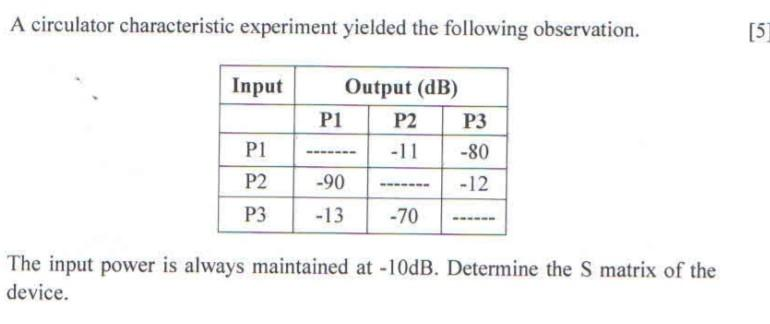Home / Expert Answers / Electrical Engineering / nbsp-a-circulator-characteristic-experiment-yielded-the-following-observation-the-input-power-pa757

# (Solved):   A circulator characteristic experiment yielded the following observation. The input power ...A circulator characteristic experiment yielded the following observation. The input power is always maintained at $$-10 \mathrm{~dB}$$. Determine the $$\mathrm{S}$$ matrix of the device.

We have an Answer from Expert